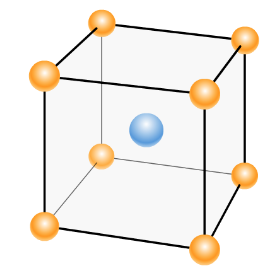# Problem: Consider a body-centered cubic unit cell as shown here.a. How many corner atoms (orange) are shown in this image?b. What fraction of each corner atom is inside the boundaries of the cube? c. How many body atoms shown in this image? d. What fraction of each body atom is inside the boundaries of the cube? e. If you sum all the fractions of atoms, how many atoms are actually inside a body-centered cubic unit cell?

###### Problem Details

Consider a body-centered cubic unit cell as shown here.

a. How many corner atoms (orange) are shown in this image?

b. What fraction of each corner atom is inside the boundaries of the cube?

c. How many body atoms shown in this image?

d. What fraction of each body atom is inside the boundaries of the cube?

e. If you sum all the fractions of atoms, how many atoms are actually inside a body-centered cubic unit cell?What scientific concept do you need to know in order to solve this problem?

Our tutors have indicated that to solve this problem you will need to apply the Unit Cell concept. You can view video lessons to learn Unit Cell. Or if you need more Unit Cell practice, you can also practice Unit Cell practice problems.

What is the difficulty of this problem?

Our tutors rated the difficulty ofConsider a body-centered cubic unit cell as shown here.a. Ho...as medium difficulty.

How long does this problem take to solve?

Our expert Chemistry tutor, Dasha took 1 minute and 49 seconds to solve this problem. You can follow their steps in the video explanation above.

What professor is this problem relevant for?

Based on our data, we think this problem is relevant for Professor Toupadakis' class at UCD.